Author: Oscar Cronquist Article last updated on November 08, 2018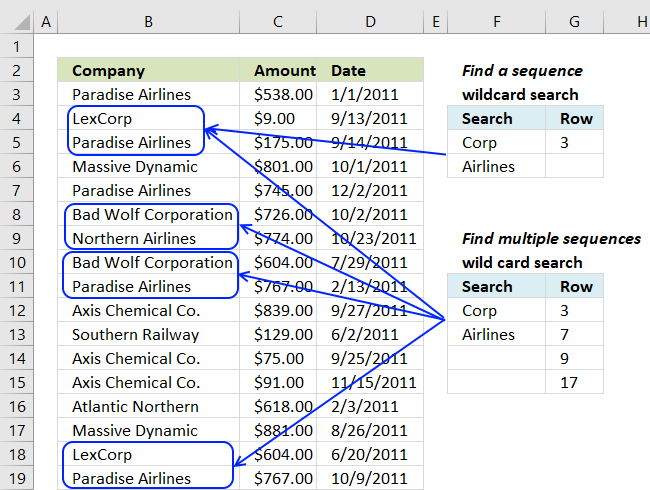In my last post I showed you how to find a sequence of values. The array formula extracted the row of the first found sequence, see below.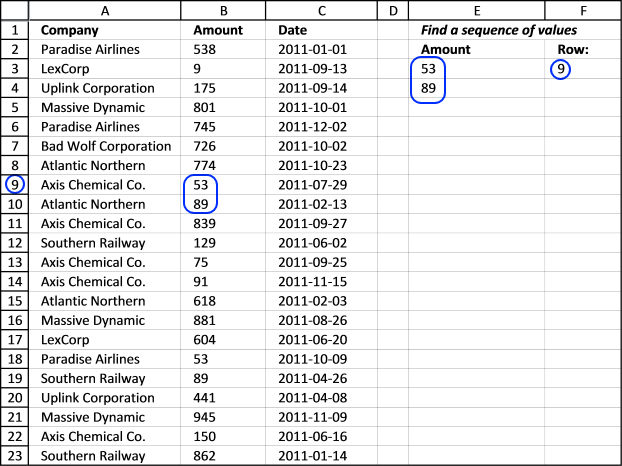Array formula in cell F3:

=MATCH(1, COUNTIFS(E3, B1:B23, E4, B2:B24), 0)

To enter an array formula, type the formula in a cell then press and hold CTRL + SHIFT simultaneously, now press Enter once. Release all keys.

The formula bar now shows the formula with a beginning and ending curly bracket telling you that you entered the formula successfully. Don't enter the curly brackets yourself.

### Explaining formula in cell F3

#### Step 1 - Create an array that shows where the specific sequence is

The COUNTIFS function lets you count values based on multiple conditions, in this case, I am also changing the cell references.

The first two arguments checks for the value in cell E3 in cell range B1:B23. The third and fourth argument looks for the value in cell E4 in cell range B2:B24, note that the cell range in argument 4 relative the 2nd argument is offset by 1.

This cell reference technique lets you find values in a given sequence.

COUNTIFS(E3,B1:B23,E4,B2:B24) returns {0; 0; 0; 0; 0; 0; 0; 0; 1; 0; 0; 0; 0; 0; 0; 0; 0; 1; 0; 0; 0; 0; 0}.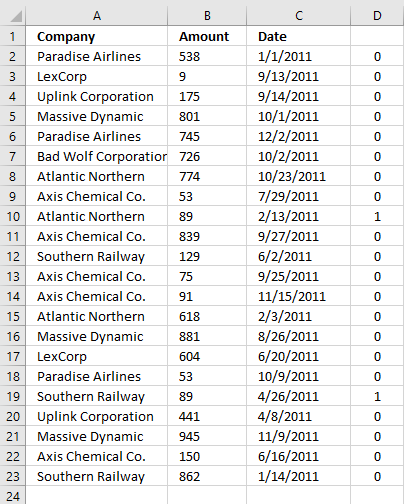The array is shown in column D.

#### Step 2 - Find the relative position in the array

The MATCH function finds the position in the array based on a given value, if multiple values exist the position of the first is returned.

MATCH(1, COUNTIFS(E3, B1:B23, E4, B2:B24), 0)

becomes

MATCH(1, {0; 0; 0; 0; 0; 0; 0; 0; 1; 0; 0; 0; 0; 0; 0; 0; 0; 1; 0; 0; 0; 0; 0}, 0)

and returns 9 in cell F3.

Recommended article

Find a sequence

This array formula finds the sequence "Axis Chemical Co." (cell E2) and "Southern Railway" (cell E3) in two consecutive cells […]

What I didn't show you was how to find multiple sequences with this particular array formula.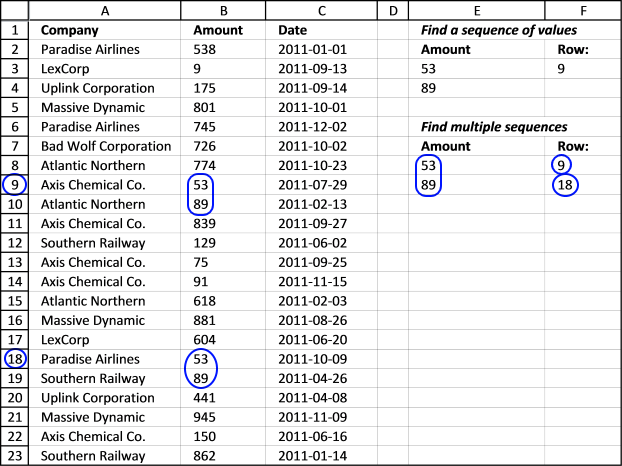This array formula finds all instances of a sequence. It returns their starting row number.

Array formula in cell F8:

=SMALL(IF(COUNTIFS(\$E\$8, \$B\$1:\$B\$23, \$E\$9, \$B\$2:\$B\$24)=1, MATCH(ROW(\$A\$1:\$A\$23), ROW(\$A\$1:\$A\$23)), ""), ROW(A1))

To enter an array formula, type the formula in a cell then press and hold CTRL + SHIFT simultaneously, now press Enter once. Release all keys.

The formula bar now shows the formula with a beginning and ending curly bracket telling you that you entered the formula successfully. Don't enter the curly brackets yourself.

### Explaining formula in cell F8

See step 1 in the explanation above before reading the rest below.

#### Step 2 - Convert boolean value TRUE to row number in array

The IF function uses a logical expression in the first argument to determine if the second or third argument is being returned. The second argument if the logical expression evaluates to TRUE and the third argument if the logical expression evaluates to FALSE.

IF(COUNTIFS(\$E\$8, \$B\$1:\$B\$23, \$E\$9, \$B\$2:\$B\$24)=1, MATCH(ROW(\$A\$1:\$A\$23), ROW(\$A\$1:\$A\$23)), "")

becomes

IF({0; 0; 0; 0; 0; 0; 0; 0; 1; 0; 0; 0; 0; 0; 0; 0; 0; 1; 0; 0; 0; 0; 0}, MATCH(ROW(\$A\$1:\$A\$23), ROW(\$A\$1:\$A\$23)), "")

becomes

IF({0; 0; 0; 0; 0; 0; 0; 0; 1; 0; 0; 0; 0; 0; 0; 0; 0; 1; 0; 0; 0; 0; 0}, {1; 2; 3; 4; 5; 6; 7; 8; 9; 10; 11; 12; 13; 14; 15; 16; 17; 18; 19; 20; 21; 22; 23}, "")

and returns

{"";"";"";"";"";"";"";"";9;"";"";"";"";"";"";"";"";18;"";"";"";"";""}

#### Step 3 - Extract row numbers sorted smallest to largest

The SMALL function extracts the k-th smallest number in array, it ignores blanks.

SMALL(IF(COUNTIFS(\$E\$8, \$B\$1:\$B\$23, \$E\$9, \$B\$2:\$B\$24)=1, MATCH(ROW(\$A\$1:\$A\$23), ROW(\$A\$1:\$A\$23)), ""), ROW(A1))

becomes

SMALL({"";"";"";"";"";"";"";"";9;"";"";"";"";"";"";"";"";18;"";"";"";"";""}, ROW(A1))

The ROW function returns the row number based on a cell reference, this cell reference is relative meaning it will change as the formula is copied to cells below. This makes the formula extracting a new value in each cell.

SMALL({"";"";"";"";"";"";"";"";9;"";"";"";"";"";"";"";"";18;"";"";"";"";""}, ROW(A1))

becomes

SMALL({"";"";"";"";"";"";"";"";9;"";"";"";"";"";"";"";"";18;"";"";"";"";""}, 1)

returns 9 in cell F8.

Recommended article

Find the longest/shortest consecutive sequence of a value

The array formula in cell D3 returns the the length of longest consecutive sequence of a value in column A.  Cell […]

### Look for a multi-level sequence

The next array formula looks for a consequtive values in columns and rows. The picture explains it all.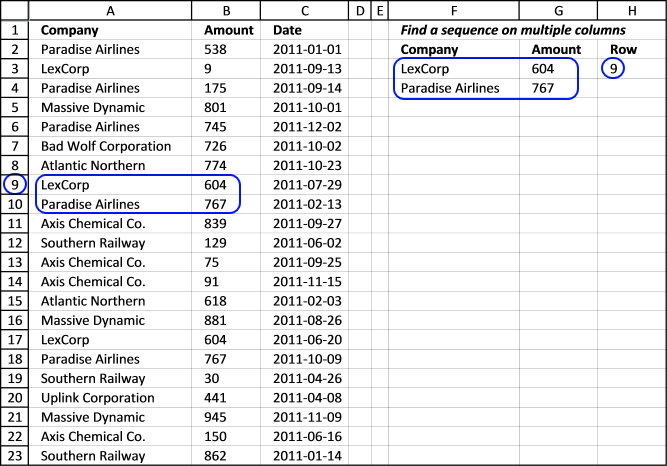Array formula in cell H3:

=MATCH(1,COUNTIFS(F3, A1:A23, F4, A2:A24, G3, B1:B23, G4,B2:B24), 0)

Use this formula if you are looking for the first instance of a sequence. It looks for a specific sequence (LexCorp and Paradise Airlines) in column A and for 604 and 767 in column B. LexCorp and 604 must be on the same row and Paradise Airlines and 767 must be on the next row below.

### Explaining formula in cell H3

#### Step 1 - Create an array that shows where the specific sequence is

The COUNTIFS function lets you count values based on multiple conditions, in this case, I am also doing changes to the cell references.

COUNTIFS(F3, A1:A23, F4,A2:A24, G3, B1:B23, G4, B2:B24) returns {0; 0; 0; 0; 0; 0; 0; 0; 1; 0; 0; 0; 0; 0; 0; 0; 1; 0; 0; 0; 0; 0; 0}.

The second record (cell F4 and G4) are offset 1 row in order to find two consecutive records.

#### Step 2 - Find the relative position in the array

The MATCH function finds the position in the array based on a given value if multiple values exist the position of the first is returned.

MATCH(1, COUNTIFS(F3, A1:A23, F4,A2:A24, G3, B1:B23, G4, B2:B24), 0)

becomes

MATCH(1, {0; 0; 0; 0; 0; 0; 0; 0; 1; 0; 0; 0; 0; 0; 0; 0; 1; 0; 0; 0; 0; 0; 0}, 0)

and returns 9 in cell H3.

Recommended article

Repeat values

### Look for multiple multi-level sequencesArray formula in cell H8:

=SMALL(IF(COUNTIFS(\$F\$8, \$A\$1:\$A\$23, \$F\$9, \$A\$2:\$A\$24, \$G\$8, \$B\$1:\$B\$23, \$G\$9, \$B\$2:\$B\$24)=1, MATCH(ROW(\$A\$1:\$A\$23), ROW(\$A\$1:\$A\$23)), ""), ROW(A1))

It is like the formula above but it returns the location of multiple sequences.

### Explaining formula in cell H8

See step 1 in the explanation above before reading the rest below.

#### Step 2 - Convert boolean value TRUE to row number in array

The IF function uses a logical expression in the first argument to determine if the second or third argument is being returned. The second argument if the logical expression evaluates to TRUE and the third argument if the logical expression evaluates to FALSE.

IF(COUNTIFS(\$F\$8, \$A\$1:\$A\$23, \$F\$9, \$A\$2:\$A\$24, \$G\$8, \$B\$1:\$B\$23, \$G\$9, \$B\$2:\$B\$24)=1, MATCH(ROW(\$A\$1:\$A\$23), ROW(\$A\$1:\$A\$23)), "")

becomes

IF({FALSE; FALSE; FALSE; FALSE; FALSE; FALSE; FALSE; FALSE; TRUE; FALSE; FALSE; FALSE; FALSE; FALSE; FALSE; FALSE; TRUE; FALSE; FALSE; FALSE; FALSE; FALSE; FALSE}, MATCH(ROW(\$A\$1:\$A\$23), ROW(\$A\$1:\$A\$23)), "")

becomes

IF({FALSE; FALSE; FALSE; FALSE; FALSE; FALSE; FALSE; FALSE; TRUE; FALSE; FALSE; FALSE; FALSE; FALSE; FALSE; FALSE; TRUE; FALSE; FALSE; FALSE; FALSE; FALSE; FALSE}, {1; 2; 3; 4; 5; 6; 7; 8; 9; 10; 11; 12; 13; 14; 15; 16; 17; 18; 19; 20; 21; 22; 23}, "")

and returns

{"";"";"";"";"";"";"";"";9;"";"";"";"";"";"";"";"";17;"";"";"";"";""}.

#### Step 3 - Extract row numbers sorted smallest to largest

The SMALL function extracts the k-th smallest number in array, it ignores blanks.

SMALL(IF(COUNTIFS(\$F\$8, \$A\$1:\$A\$23, \$F\$9, \$A\$2:\$A\$24, \$G\$8, \$B\$1:\$B\$23, \$G\$9, \$B\$2:\$B\$24)=1, MATCH(ROW(\$A\$1:\$A\$23), ROW(\$A\$1:\$A\$23)), ""), ROW(A1))

becomes

SMALL({"";"";"";"";"";"";"";"";9;"";"";"";"";"";"";"";17;"";"";"";"";"";""}, ROW(A1))

The ROW function returns the row number based on a cell reference, this cell reference is relative meaning it will change as the formula is copied to cells below. This makes the formula extracting a new value in each cell.

SMALL({"";"";"";"";"";"";"";"";9;"";"";"";"";"";"";"";17;"";"";"";"";"";""}, ROW(A1))

becomes

SMALL({"";"";"";"";"";"";"";"";9;"";"";"";"";"";"";"";17;"";"";"";"";"";""}, 1)

returns 9 in cell F8.

Recommended article

Locate lookup values in a table [HYPERLINK]

Today I´ll show you how to search a table column and jump to that table cell using the hyperlink function. When […]

### Wild card search for a sequence

This demonstrates how to do a wild card search for a sequence. This formula returns the row number of first found sequence in column A. The formula looks for a text string in a cell and another text string in the cell below. You don´t need to enter the wild cards * (asterisks), see cell F3 and F4.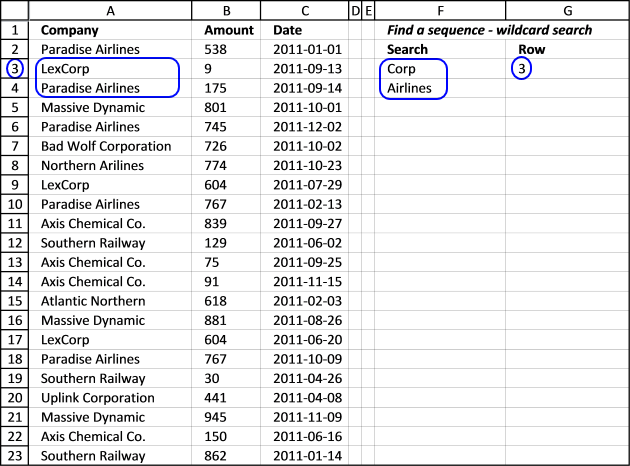=MATCH(TRUE, ISNUMBER(FIND(\$F\$11, \$A\$1:\$A\$23)*FIND(\$F\$12, \$A\$2:\$A\$24)), 0)

insertexcerpt id="1450"]

### Wild card search for multiple sequences

This demonstrates how to do wild card searches for multiple sequences. This formula returns the row number of all found sequences in column A. The sequence is in cell F11 and F12.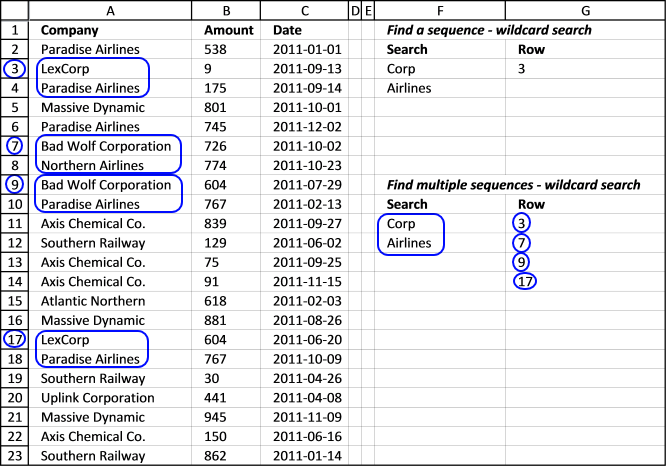Array formula in cell G11:

=SMALL(IF(ISNUMBER(FIND(\$F\$11, \$A\$1:\$A\$23)*FIND(\$F\$12, \$A\$2:\$A\$24)), MATCH(ROW(\$A\$1:\$A\$23), ROW(\$A\$1:\$A\$23)), ""), ROW(A1))

If a cell contains "Corp" and the next cell beneath contains "Airlines" the array formula returns the row number.

Search for multiple text strings in column – AND logic

Question: How do I search a list for two text strings and return a list with where both strings occurs? […]

Find a sequence of values.xlsx

### Functions in this post

How to use the IF function

Checks if a logical expression is met. Returns a specific value if TRUE and another specific value if FALSE.

How to use the SMALL function

The SMALL function lets you extract a number in a cell range based on how small it is compared to the other numbers in the group.

How to use the MATCH function

Identify the position of a value in an array.

How to use the COUNTIFS function

Checks multiple conditions against the same number of cell ranges and counts how many times all criteria are met.

How to use the SEARCH function

Returns a number representing the position a substring is, if found in a textstring. FIND is case sensitive and SEARCH is not.